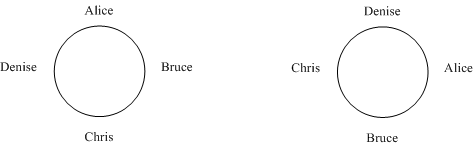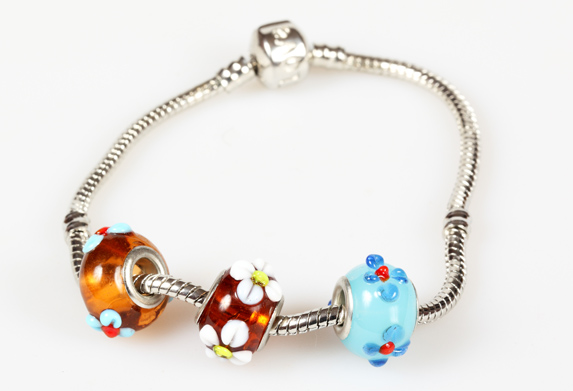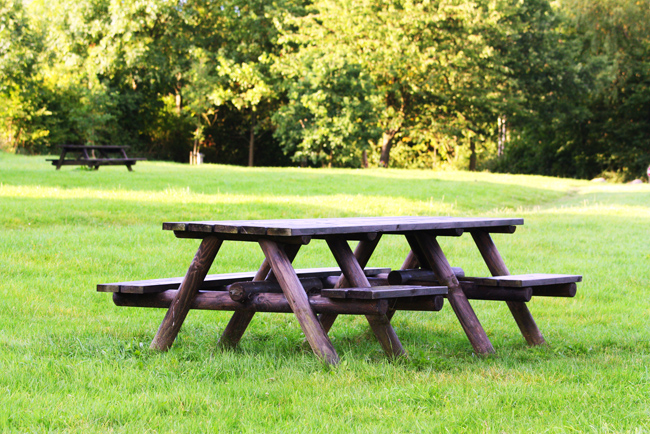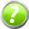x2
Introduction
( )
Types of Arrangements
=
Two More Methods
=
Some Applications

# Section 1.8 – Circles and Repetition

Suppose you went out to dinner with three friends and the four of you were seated at a table. How many different possible seating arrangements are there?

The order that you sit matters here so this is an example of a permutation. At this point, you might be tempted to pull out the permutation formula and say that there are 4! or 24 but there's a problem with that number. The permutation formula counts {Alice, Bruce, Chris, Denise} as different from {Denise, Alice, Bruce, Chris} but look at what happens if I draw those two arrangements around a circular table.Are those really different? If you think about who is sitting to the left and right of each person, you'll see that everyone is sitting next to the same people in both arrangements. For example, in both tables Alice has Bruce on her left and Denise on her right. If we start with an arrangement like {Alice, Bruce, Chris, Denise} then every rotation of the table gives us an identical result. Think of it is everyone getting up and moving to the seat on their right, like a game of musical chairs. Because every arrangement has four "rotated" versions around the table our number of arrangements is off by a factor of four which means the actual number is 24 / 4 = 6.

Permutations around a Circle

The number of permutations of n distinct objects placed in a circle is

$$\frac{n!}{n}= (n - 1)!$$

# Example 1 – Arrangements in a CircleHow many ways can 7 different charms be arranged on a charm bracelet?

The charms are different so we can apply the circular permutation formula to conclude that there are (7 - 1)! or 6! = 720 different arrangements.

# Example 2 – Seating Groups at a TableTen people are being seated at a table. Four of them are kids who want to sit together. How many ways can the group be seated?

With this kind of question, it helps to think of the kids asked one block. This means we have to arrange six adults and one group of kids around the table. That's a total of seven "objects" so the circular permutation formula tells us that there are 6! possible arrangements.

For every one of those 720 arrangements, the kids in their group can be seated in 4! different ways. The multiplication rule tells us that the total number of arrangements must be

6! · 4! = 720 · 24 = 17, 280

Example 2 illustrates a common approach to counting problems. You start by taking a subgroup (the kids) within a larger group (the entire table) and treat the subgroup as one object. Now, counting the number of arrangements involves two choices:

1. Choose an arrangement of the objects.
2. Choose an arrangement of the subgroup.

If you can count the number of ways that you can make those two choices, then you can get the total number of choices using the Multiplication Rule. In Example 2, the two steps were

(choose an arrangement of the adults and the kids' group)and(choose an arrangement of the kids in the group)

Once we had the number of ways that those two choices could be made, the Multiplication Rule gave us the final result

(total seating arrangements) = (arrangements of the adults and the kids' group) · (arrangements of the kids' group)

One other thing you should notice about Example 2, is that I didn't use the circular permutation formula to find the number of ways that the kids could be arranged. I did this because the kids are being seated in a section of the circle rather than a complete circle.

# Example 3 – Circular Arrangements

How many ways can the numbers -3, -2, -1, 0, 1, 2, 3 be arranged in a circle if two of the negative numbers have to be together?

This question is similar to Example 2. First, count the number of ways that the 3 negative numbers can be arranged in a group of 2 then count the way that the remaining 5 numbers plus the negative number group can be arranged in a circle.

(total) = (permutations of the neg. numbers) · (permutations of the remaining numbers and the one neg. number group)

The 3 negative numbers can be arranged in a group of 2 in 3P2 or 3! / (3 - 2)! = 6 ways. The circular permutation formula tells us that the 5 remaining numbers plus the group of two negative numbers can be arranged in a circle in (6 - 1)! = 5! = 120 ways. That gives us

(total) = 6 · 120 = 720

## Identical Objects

All of the situations that we've discussed so far have assumed that all of the things be arranged are unique, for example people or charms on a bracelet. That's not always going to be the case. For example, suppose you wanted to count the number of words that you can make from the letters ARRANGER. Our permutation formula would treat all three R's as different letters to RARANGER would get counted a total of six times, e.g. R1AR2ANGER and R2AR1ANGER. (R1 is the first R in ARRANGER and R2 is the second. I added them so you could see the difference.) We can get the correct number of arrangements using the Multiplication Rule.

First, we can assign the 8 available positions to the 3 R's in 8C3 ways.
Second, we can assign the remaining 5 positions to the 2 A's in 5C2 ways.
Third, the can assign the 3 remaining positions to the 1 N in 3C1 ways.
The 2 remaining positions can be assigned to the G in 2C1 ways.
Finally, the 1 remaining position can be assigned to the E in 1C1 ways.

According to the Multiplication Rule, we can get the total number of arrangements by multiplying all of those individual choices together:

$$_8C_3 \cdot _5C_2 · _3C_1 · _2C_1 · _1C_1=\frac{8!}{3!5!}\frac{5!}{2!3!}\frac{3!}{1!2!}\frac{2!}{1!1!}\frac{1!}{1!0!} = \frac{8!}{3!2!2!1!1!} = 1,160$$

If the way I canceled going to the second line seems a little odd then take a close look at the bottom numbers: 3, 2, 2, 1 and 1. Notice that those are the number of times that R, A, N, G and E appear in the original word.

Permutations with Identical Objects

The number of different permutations of n objects where there are n1 identical copies of one object, n2 identical copies of a second object, . . . and nk copies of another object is

$$\frac{n!}{n_1! n_2! n_3! . . .n_k!}$$

If that seems a little confusing, just think back to our example. The numerator is just the factorial of the total number of objects (the eight letters in our example) and the numbers in the denominator are just the number of times that each letter appears in the original word.

# Example 4 – Identical Objects

How many six digit numbers can be formed using the digits 1, 1, 1, 3, 3 and 4?

In our number, there will be 3 one's, 2 three's and 1 four. Substituting those values into the permutations with identical objects formula gives us

$$\frac{6!}{3!2!1!}=\frac{720}{12}= 60$$

So there are a total of 60 possible six digit numbers.

# Example 5 – Identical Objects

How many ways are there to arrange the letters in the word STATISTICS if the two I's have to be together?

We can treat the two I's as a single letter which gives us these counts for each letter:

S:  3T:  3A:  1II:  1C:  1

Substituting those values into the permutations with identical objects formula gives us

$$\frac{9!}{3!3!1!1!1!}=\frac{362880}{36}= 10, 080$$

So there are a total of 10,080 possible arrangements.

# Exercises

### Exercises= show solution= get hint

1. If your dinner table has room for six chairs, how many different ways can six people be seated at the table?
2. Suppose you get a new key ring and you have five keys that you have to put on it. How many different ways can you arrange the keys?
3. A group of 4 adults, 2 teenagers and 3 younger kids are being seated together at a restaurant. How many different seating arrangements are there if the teenagers want to sit together and the younger kids want to sit together?
4. How many ways can the letters {A, B, C, D, e, f, g, h} be arranged in a circle if exactly three of the lower case letters have to be together?
5. How many ways can the letters {A, B, C, D, e, f, g, h} be arranged in a circle if at least three of the lower case letters have to be together?
6. Eight couples are sitting together at a table. If each couple has to sit together then how many seating arrangements are there?
7. How many six letter words can be made from the letters in HAWAII if each letter has to be used once?
8. A carpenter is designing wood ornaments for a Christmas display. The ornaments are made up of alternating pieces of three different kinds of wood. If each ornament has two pieces of each type of wood and the artist wants to make each piece different, how many different ornaments can he make? (Assume the pieces of wood are being laid in a straight line.)
9. How many eight digit numbers can be made from the numbers 1, 2, 3, 3, 3, 5, 7 and 8?
10. How many eight digit numbers can be made from the numbers 1, 2, 3, 3, 3, 5, 7 and 8 if the first number has to be a 1?
11. How many eight digit numbers can be made from the numbers 1, 2, 3, 3, 3, 5, 7 and 8 if the 2 has to be followed by a 3?

### Analysis

1. In our discussion of circular arrangements, we only talked about permutations. Explain why we don't need a formula for "circular combinations".
2. Look back at your answer to question seven. Suppose you have to arrange n distinct objects, e.g. the people, in a circle along with r identical objects, e.g. the empty chairs. Come up with a formula for the total number of arrangements.

### Technical Writing

1. Explain why the regular permutation formula doesn't work for permutations in a circle.
2. Explain the reasoning behind your solution to question six.